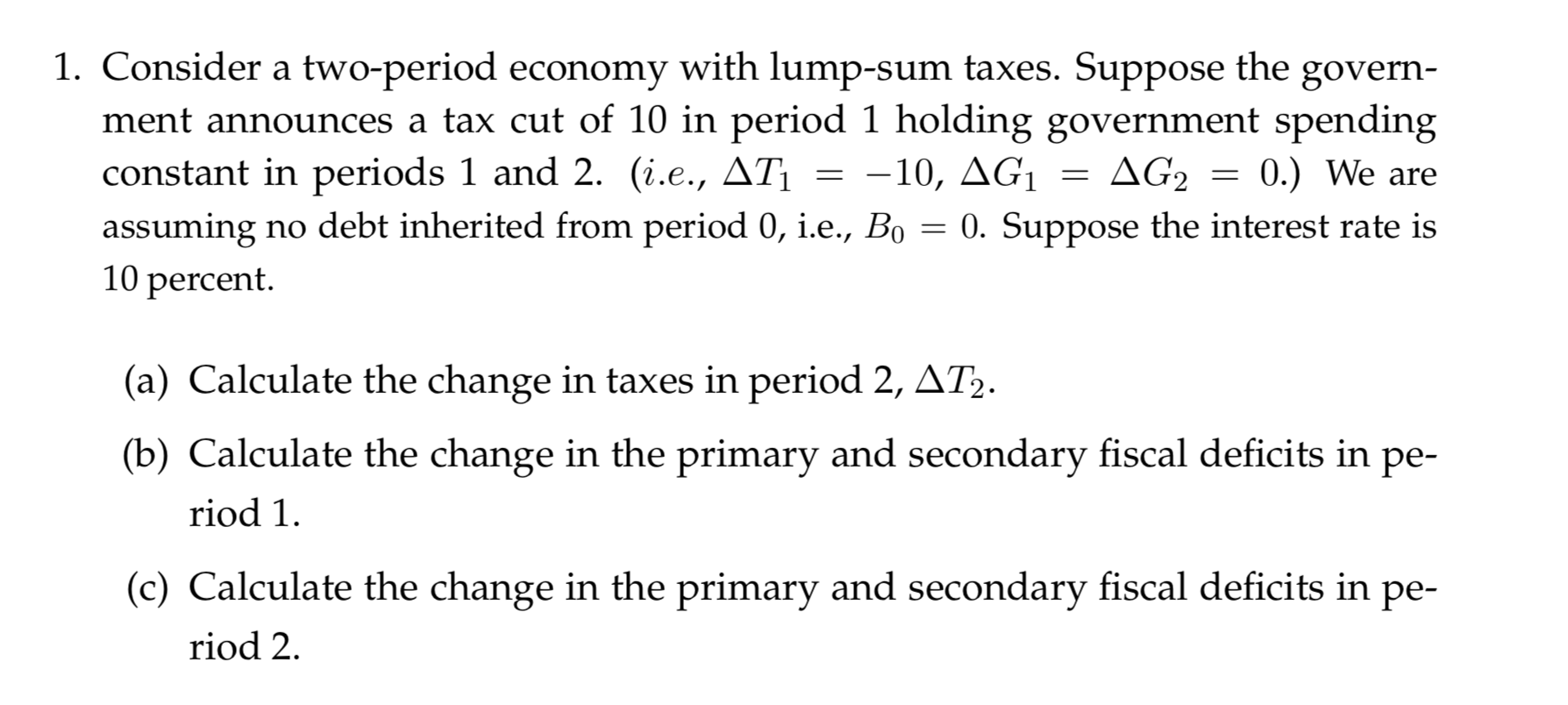# 1. Consider a two-period economy with lump-sum taxes. Suppose thement announces a tax cut of 10 in period 1 holding government spendingconstant in periods 1 and 2. (i.e., ATassuming no debt inherited from period 0, i.e., Bo10 percentgovernΔG,0.) We are-10, AG10. Suppose the interest rate is(a) Calculate the change in taxes in period 2, AT2.(b) Calculate the change in the primary and secondary fiscal deficits inре-riod 1(c) Calculate the change in the primary and secondary fiscal deficits in pe-riod 2

Question
153 views

I need help with parts a, b and chelp_outlineImage Transcriptionclose1. Consider a two-period economy with lump-sum taxes. Suppose the ment announces a tax cut of 10 in period 1 holding government spending constant in periods 1 and 2. (i.e., AT assuming no debt inherited from period 0, i.e., Bo 10 percent govern ΔG, 0.) We are -10, AG1 0. Suppose the interest rate is (a) Calculate the change in taxes in period 2, AT2. (b) Calculate the change in the primary and secondary fiscal deficits in ре- riod 1 (c) Calculate the change in the primary and secondary fiscal deficits in pe- riod 2 fullscreen
check_circle

Step 1

In the two period economy with government and lump sum tax, we have the following budget constraints of the government

Step 2

To get the lifetime budget constraint of the government combine the two equations: ie solving equation gives us:

Step 3
1. A) The government has to satisfy it’s lifetime budget constraint and that means that the amount of tax in period 2 changes by the following

&n...

### Want to see the full answer?

See Solution

#### Want to see this answer and more?

Solutions are written by subject experts who are available 24/7. Questions are typically answered within 1 hour.*

See Solution
*Response times may vary by subject and question.
Tagged in